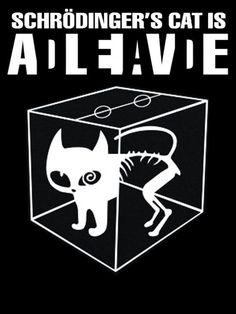$$\renewcommand{\vec}{\boldsymbol{\mathrm{#1}}}$$Continuing something I began about a month ago, I spent more of my free time than I care to admit re-deriving some of the most basic identities in quantum physics.I started with the single-particle case of a harmonic oscillator. Such an oscillator is characterized by the classical Lagrangian

$$L=\frac{1}{2}m\dot{\vec{q}}^2-\frac{1}{2}k\vec{q}^2-V(\vec{q}),$$

and the corresponding Hamiltonian

$$H=\frac{\vec{p}^2}{2m}+\frac{1}{2}k\vec{q}^2+V(\vec{q}).$$

By multiplying this Hamiltonian with $$\psi=e^{i(\vec{p}\cdot\vec{q}-Ht)/\hbar}$$, we basically obtain Schrödinger’s equation:

$$\left[i\hbar\partial_t+\frac{\hbar^2}{2m}\vec{\nabla}^2-\frac{1}{2}k\vec{q}^2-V(\vec{q})\right]e^{i(\vec{p}\cdot\vec{q}-Ht)/\hbar}=0.$$

The transition to the quantum theory begins when we accept that linear combinations of solutions of this equation (i.e., $$\psi$$-s corresponding to different values of $$\vec{p}$$ and $$H$$) also represent physical states of the system, despite the fact that these “mixed” solutions are not eigenfunctions and there are no corresponding classical eigenvalues $$\vec{p}$$ and $$H$$.

Pure algebra can lead to an expression of $$\hat{H}$$ in the form of “creation” and “annihilation” operators:

$$\hat{H}=\hbar\omega\left(\hat{a}^\dagger\hat{a}+\frac{1}{2}\right)+V(\vec{q}).$$

These operators have the properties

\begin{align*}
\hat{H}\hat{a}\psi_n&=\left([\hat{H},\hat{a}]+\hat{a}\hat{H}\right)\psi_n=(E_n-\hbar\omega)\hat{a}\psi_n,\\
\hat{H}\hat{a}^\dagger\psi_n&=\left([\hat{H},\hat{a}^\dagger]+\hat{a}^\dagger\hat{H}\right)\psi_n=(E_n+\hbar\omega)\hat{a}^\dagger\psi_n.
\end{align*}
where

$$E_n=\left(n+\frac{1}{2}\right)\hbar\omega.$$

This same derivation can be done in the relativistic single particle case as well.

Moreover, it is possible to define a classical scalar field in the form

$${\cal L}=\frac{1}{2}\rho(\partial_t\phi)^2-\frac{1}{2}\rho c^2(\vec{\nabla}\phi)^2-\frac{1}{2}\kappa\phi^2-V(\phi),$$

which leads to the Hamiltonian density

$${\cal H}=\pi\partial_t\phi-{\cal L}=\frac{\pi^2}{2\rho}+\frac{1}{2}\rho c^2(\vec{\nabla}\phi)^2+\frac{1}{2}\kappa\phi^2+V(\phi).$$

The transitioning to the quantum theory occurs by first expressing $$\phi$$ as a Fourier integral and then promoting the Fourier coefficients to operators that satisfy a commutation relation in the form

$$[\hat{a}(\omega,\vec{k}),\hat{a}^\dagger(\omega,\vec{k}’)]=(2\pi)^3\delta^3(\vec{k}-\vec{k}’).$$

This leads to a commutation relation for the field and its canonical momentum in the form

$$[\hat{\phi}(t,\vec{x}),\hat{\pi}(t,\vec{x}’)]=i\hbar\delta^3(\vec{x}-\vec{x}’),$$

and for the Hamiltonian,

$$\hat{H}=\hbar\omega\left\{\frac{1}{2}+\int\frac{d^3\vec{k}}{(2\pi)^3}\hat{a}^\dagger(\omega,\vec{k})\hat{a}(\omega,\vec{k})\right\}+\int d^3xV(\hat{\phi}).$$

More details are provided on my Web site, at https://www.vttoth.com/CMS/physics-notes/297.

So why did I find it necessary to capture here something that can be found in first chapter of every semi-decent quantum field theory textbook? Several reasons.

• First, I wanted to present a consistent treatment of all four cases: the nonrelativistic and relativistic case for both the particle and the field theory.
• Second, I wanted to write down all relevant equations without omitting dimensions. I wanted to write down a Lagrangian density that has the dimensions of energy density, consistent with a scalar field that has the dimensions of length (i.e., a displacement).
• Third, I wanted to spell out some of the details of the derivation that are omitted from nearly all textbooks yet, I am obliged to admit, almost stumped me. That is, once you see the derivation the steps are reasonably trivial, but it is still hard to stumble upon exactly the right way to apply the relevant identities related to Fourier transforms and Dirac deltas.
• Lastly, I find it revealing how this approach can highlight exactly where a quantum theory is introduced. In the particle theory case, it is when we assume that “mixed states”, that is, linear combinations of eigenstates also represent physical states of a system, despite the fact that they do not correspond to classical eigenvalues. In the case of a field theory, the transition occurs when we replace Fourier coefficients with operators: implicit in the transition is that once again, mixed states are included as representing actual physical states of the system.

Note also how none of this has anything to do with interpretations. There is no “collapse of the wave function” or any such nonsense. That stuff happens when we introduce into our consideration a “measurement event”, effectively an interaction between the quantum system and a classical instrument, which forces the quantum system into an eigenstate. This eigenstate cannot be predicted from the initial conditions alone, precisely because the classical idealization of the measurement apparatus effectively amounts to an admission of ignorance about its true quantum state.